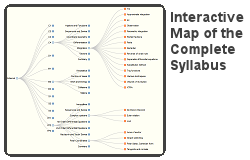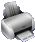Go to content

## 815 pages

84
N: Structure and Calculation
18
N: Fractions, Decimals and Percentages
38
N: Measures and Accuracy
119
A: Notation, Vocabulary and Manipulation
121
A: Graphs
171
A: Solving Equations and Inequalities
23
A: Sequences
17
R: Ratio, Proportion and Rates of Change
65
G: Properties and Constructions
93
G: Mensuration and Calculation
10
G: Vectors
10
P: Probability
46
S: Statistics

## Study Online

Study Edexcel GCSE (9-1) Higher (UK) online. There are currently 815 pages of content ranging from illustrations and explanations to 374 fully worked exam questions and 202 o-tests (on-line assessments). Almost every page is interactive offering you much more than simply an online text book.

## Assessment Content

O-tests (Online Tests) arranged in levels as follows:

## FeaturesPrint out exam papers based on past exam papers.

There is extensive online help available for assisting with subjects such as:

• handling your account(s) - for schools how to set up and use separate teacher and student personal accounts;
• setting up student classes and accounts, including importing data from external sources;
• assessment from both the student's and teacher's perpective (including, for teachers, how to create and allocate student tasks);
• how to use o-test progress charts;
• how teachers can monitor the progress of their students;
• setting up and printing exam papers (including how to handle common printing problems).

To access the online help click or touch the button near the top-right of the page. This button will appear with a green background when there is help available directly related to the page currently displayed.

ID 14072: A18: Solving Quadratics by Completing the Square and Quadratic Formula : Equation Buster : O-test 7
Edexcel GCSE (9-1) Higher (UK) A: Solving Equations and Inequalities

ID 14070: A18: Solving Quadratics by Completing the Square and Quadratic Formula : Equation Buster : O-test 6
Edexcel GCSE (9-1) Higher (UK) A: Solving Equations and Inequalities

ID 14069: A18: Solving Quadratics by Completing the Square and Quadratic Formula : Equation Buster : O-test 5
Edexcel GCSE (9-1) Higher (UK) A: Solving Equations and Inequalities

ID 14068: A18: Solving Quadratics by Completing the Square and Quadratic Formula : Equation Buster : O-test 4
Edexcel GCSE (9-1) Higher (UK) A: Solving Equations and Inequalities

ID 14067: A18: Solving Quadratics by Completing the Square and Quadratic Formula : Equation Buster : O-test 3
Edexcel GCSE (9-1) Higher (UK) A: Solving Equations and Inequalities

ID 14061: A18: Solving Quadratics by Completing the Square and Quadratic Formula : Equation Buster : Level 2b
Edexcel GCSE (9-1) Higher (UK) A: Solving Equations and Inequalities

ID 14058: A18: Solving Quadratics by Completing the Square and Quadratic Formula : Equation Buster : O-test 2
Edexcel GCSE (9-1) Higher (UK) A: Solving Equations and Inequalities

ID 14057: A18: Solving Quadratics by Completing the Square and Quadratic Formula : Equation Buster : O-test 1
Edexcel GCSE (9-1) Higher (UK) A: Solving Equations and Inequalities

ID 14056: A18: Solving Quadratics by Completing the Square and Quadratic Formula : Equation Buster : Level 8
Edexcel GCSE (9-1) Higher (UK) A: Solving Equations and Inequalities

ID 14055: A18: Solving Quadratics by Completing the Square and Quadratic Formula : Equation Buster : Level 7
Edexcel GCSE (9-1) Higher (UK) A: Solving Equations and Inequalities

ID 14054: A18: Solving Quadratics by Completing the Square and Quadratic Formula : Equation Buster : Level 6
Edexcel GCSE (9-1) Higher (UK) A: Solving Equations and Inequalities

ID 14050: A4: Further Algebraic Manipulation : Rational Expressions : Example 8
Edexcel GCSE (9-1) Higher (UK) A: Notation, Vocabulary and Manipulation

How exam papers are marked
Get advice from examiners and some tips on improving your exam technique - particularly how to avoid zero marks!
Mathematicians
Read brief biographies of famous Mathematicians who were instrumental in creating advanced mathematics.
Interactive glossary
Check the meaning of words and phrases used throughout your course. A relevant glossary also appears on each page.